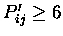Next: Output to standard output Up: Input and Output format Previous: Multiple alignment format

# Output from SCANS

Output from STAMP scans consists of a list of domains and a corresponding set of scores, lengths and other numbers that can be used to sort and understand the output.

The format is as follows (see examples/1cmsN_stamp_scan.trans):

% Output from STAMP scanning routine
%
% Domain 1cmsN was used to scan the domain database:
%  ac_prot.domains
% 2 fits were performed
% Fit 1 E1= 20.000, E2=  3.800, CUT=  1.000
% Fit 2 E1=  3.800, E2=  3.800, CUT=  4.500
% Approximate fits (alignment from N-termini) were performed
%   at every 5 residue of the database sequences
% Transformations were output for Sc=  2.000
%
% Domain used to scan
# Sc= 10.000 RMS=  0.01  Len= 999 nfit= 999 Seqid= 100.00 Secid= 100.00 q_len=  175 d_len=  175
n_sec= 100 n_equiv 999 fit_pos= _  0 _
/disk3/pdb/pdb1cms.ent 1cmsN { _ 1 _ to _ 175 _  }
# Sc=   9.744 RMS=   0.000 len=  174 nfit=  174 seq_id= 99.43 sec_id= 94.86 q_len=  175 d_len=  175
n_sec=   18 n_equiv=  173 fit_pos= _   1 _
/disk3/pdb/pdb1cms.ent 1cmsN_1 {  _ 1 _ to _ 175 _
1.00000    0.00000    0.00000         0.00000
0.00000    1.00000    0.00000         0.00000
0.00000    0.00000    1.00000         0.00000  }
# Sc=   2.749 RMS=   2.352 len=  204 nfit=   63 seq_id=  8.00 sec_id= 41.14 q_len=  175 d_len=  148
n_sec=   13 n_equiv=   58 fit_pos= _ 176 _
/disk3/pdb/pdb1cms.ent 1cmsC_1 {  _ 176 _ to _ 323 _
-0.98340   -0.10624   -0.14708        36.75176
<etc.>


(note that the lines begining by #' symbols have been wrapped here) % denotes a comment, and #' denotes numbers corresponding to the domain description described below (both will be ignored by all programs except for SORTTRANS, which uses the #' fields to sort and interpret the data.

Sc' is the STAMP Score for the comparison of the query to each database sequence. RMS' is the RMS difference between equivalenced atoms, len' is the alignment length, nfit' is the number of atoms used during the final fit of the two domains, seq_id' and sec_id' are the sequence and secondary structure identities, q_len' and d_len' are the lengths of the query and database structure (in residues), n_sec' is the number of equivalenced secondary structures, and n_equiv' are the number of residues found within stretches of 3 or more having. These fields are used during any run of SORTTRANS to sort and remove redundant/poor superimpositions. fit_pos' is the brookhaven numbering of the position in the database sequence to which the query's N-terminal end was aligned for the initial fit. The transformation supplied is that for the superimposition of the database structures onto the query.Next: Output to standard output Up: Input and Output format Previous: Multiple alignment format
Geoff Barton
1999-04-16